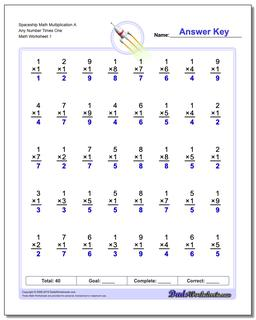One Dad. Four daughters. 9,393 worksheets... and counting!# Spaceship Math Multiplication A Any Number Times One

PropertyValue
DescriptionSpaceship Math Multiplication A Any Number Times One: These basic math fact multiplication worksheets are similar to the RocketMath, Mad Math Minutes or Mastering Math Facts multiplication programs used at many schools. These are typically one minute, timed tests (Try this countdown timer!)
Resource TypeWorksheet